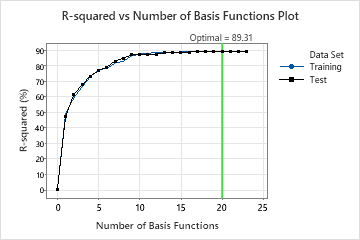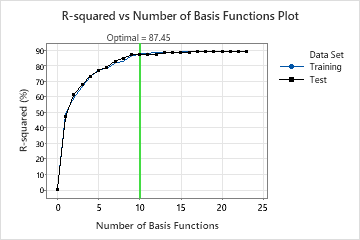# Select an alternative model from MARS® Regression

Run Predictive Analytics Module > MARS® Regression. Select Select an Alternative Model after the R-squared vs number of basis functions plot plot or the Mean absolute deviation vs number of basis functions plot plot.

## Overview

Minitab Statistical Software produces results for the model with the best value of an optimality criterion. The criterion is either the least squared error or the least absolute deviation, depending on your choice. Minitab lets you explore other models from the sequence that led to the identification of the optimal model. Typically, you select an alternative model for one of the following two reasons:
• The model that the analysis selects is part of a pattern where the criterion improves. Typically, you want to make predictions from a model with as much prediction accuracy as possible.
• The model that the analysis selects is part of a pattern where the criterion is relatively flat. One or more models with similar model summary statistics have much fewer basis functions than the optimal model. Typically, a model with fewer basis functions gives a clearer picture of how each predictor variable affects the response values. If the difference in prediction accuracy for a smaller model is negligible, you can use the smaller model to evaluate the relationships between the response and the predictor variables.
For example, the following plot accompanies results about the model with 20 basis functions. Other models in the sequence have similar R2 values.The model with 10 basis functions has an R2 value that is almost as high as the model with 20 basis functions. Typically, a model with fewer basis functions gives a clearer picture of how each predictor variable affects the response values. If the reduction in prediction accuracy from a much smaller model is negligible, you can use the much smaller model to evaluate the relationships between the response and the predictor variables.In addition to the criterion values for alternative models, you can also compare the complexity of models and the usefulness of different regions. Consider the following examples of reasons that an analyst chooses a particular model that does not sacrifice performance when compared to other models:
• The analyst chooses a smaller model that provides a clearer view of the most important variables.
• The analysis chooses a model because the basis functions are for variables that are easier to measure than the variables in another model.
• The analyst chooses a model because a particular region of the predictors is of interest.

## Perform the analysis

Select Select an Alternative Model in the output. A dialog box opens that shows a plot of the criterion against the number of basis functions and a table that summarizes the steps.

## Compare the criteria

To select an alternative model, select a point on the graph or a row in the table. Select Display results to create the results for that model.

Once you display the results, you can select Predict to make predictions from the model. For more information, go to Predict new results for MARS® Regression.

###### Tip

To compare the output of two different analyses or reports, right-click the second item in the Navigator and choose Open in Split View.